# Texas Go Math Grade 5 Lesson 2.5 Answer Key Estimate with 2-Digit Divisors

Refer to our Texas Go Math Grade 5 Answer Key Pdf to score good marks in the exams. Test yourself by practicing the problems from Texas Go Math Grade 5 Lesson 2.5 Answer Key Estimate with 2-Digit Divisors.

## Texas Go Math Grade 5 Lesson 2.5 Answer Key Estimate with 2-Digit Divisors

Unlock the Problem

The observation deck of the Willis Tower in Chicago, Illinois, is 1,353 feet above the ground. Elevators lift visitors to that level in 60 seconds. About how many feet do the elevators travel per second?Estimate. 1,353 ÷ 60
STEP 1: Use two sets of compatible numbers to find two different estimates.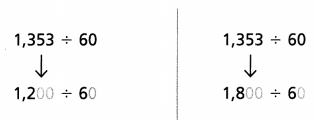STEP 2:
Use patterns and basic facts to help estimate.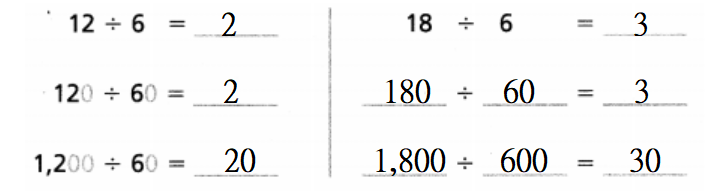Now,
The elevators travel about 20 to 30 feet per second.
The more reasonable estimate is 20 because 1,200 is closer to 1,353 than 1,800 is.
So,
The observation deck elevators in the Willis Tower travel about 20 feet per second.

Example

Estimate Money
Miriam has saved $650 to spend during her 18-day trip to Chicago. She doesn’t want to run out of money before the trip is over, so she plans to spend about the same amount each day. Estimate how much she can spend each day. Estimate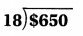$600 ÷ 20 = $30 or$800 ÷ 20 = $40 So, Miriam can spend about$30 to $40 each day. Given Miriam’s situation, which estimate do you think is the better one for her to use? Explain your reasoning. Answer: Given Miriam’s situation, The estimate of$600 is a better one for her to use because $600 is close to$650 than $800 Hence, from the above, We can conclude that Given Miriam’s situation, the estimate of$600 is a better one for her

Math Talk
Mathematical Processes

Would it be more reasonable to have an estimate or an exact answer for this example? Explain your reasoning.
From the above example,
It is given that
Miriam has saved $650 to spend during her 18-day trip to Chicago. She doesn’t want to run out of money before the trip is over, so she plans to spend about the same amount each day So, According to the given information, We can’t get the exact answer Hence, It would be more reasonable to have an estimate than an exact for the given example Try This! Use compatible numbers. Find two estimates.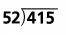Answer: The given division expression is: 415 ÷ 52 Now, Estimate: 400 ÷ 50 (or) 450 ÷ 50 = 8 (or) = 9 Now, Since 415 is close to 400, the estimate of 400 will be more reasonable Hence, from the above, We can conclude that The value of the given expression is about 8Answer: The given division expression is:$2,764 ÷ 38
Now,
Estimate:
$2,800 ÷ 40 (or)$2,400 ÷ 40
= $70 (or) =$60
Now,
Since $2,764 is close to$2,800, the estimate of $2,800 will be more reasonable Hence, from the above, We can conclude that The value of the given expression is about$70

Share and Show

Use compatible numbers to find two estimates.

Question 1.140 ÷ 20 = _________
160 ÷ 20 = _________
The given division expression is: 154 ÷ 22
Now,
Estimate:
140 ÷ 20 = 7
160 ÷ 20 = 8
Now,
Since 152 is close to 160, the estimate of 160 will be more reasonable
Hence, from the above,
We can conclude that
The value of the given expression is about 8

Question 2.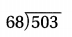The given division expression is: 503 ÷ 68
Now,
Estimate:
500 ÷ 50 = 100
560 ÷ 70 = 8
Now,
Since 68 is close to 70, the estimate of 560 will be more reasonable
Hence, from the above,
We can conclude that
The value of the given expression is about 8

Question 3.The given division expression is: 7,052 ÷ 81
Now,
Estimate:
7,200 ÷ 80 = 90
6,400 ÷ 80 = 80
Now,
Since 7,200 is close to 7,052, the estimate of 7,200 will be more reasonable
Hence, from the above,
We can conclude that
The value of the given expression is about 90

Question 4.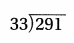The given division expression is: 291 ÷ 33
Now,
Estimate:
300 ÷ 30 = 10
270 ÷ 30 = 9
Now,
Since 300 is close to 291, the estimate of 300 will be more reasonable
Hence, from the above,
We can conclude that
The value of the given expression is about 10

Question 5.The given division expression is: 2,365 ÷ 58
Now,
Estimate:
2,400 ÷ 60 = 40
2,500 ÷ 50 = 50
Now,
Since 2,365 is close to 2,400, the estimate of 2,400 will be more reasonable
Hence, from the above,
We can conclude that
The value of the given expression is about 40

Question 6.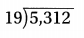The given division expression is: 5,312 ÷ 19
Now,
Estimate:
5,000 ÷ 20 = 250
5,500 ÷ 20 = 275
Now,
Since 5,000 is close to 5,312, the estimate of 5,000 will be more reasonable
Hence, from the above,
We can conclude that
The value of the given expression is about 250

Problem Solving

Use compatible numbers to estimate the quotient.

Question 7.The given division expression is: 228 ÷19
Now,
Estimate:
220 ÷ 20 = 11
240 ÷ 20 = 12
Now,
Since 220 is close to 228, the estimate of 220 will be more reasonable
Hence, from the above,
We can conclude that
The value of the given expression is about 11

Question 8.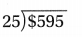The given division expression is: $595 ÷ 25 Now, Estimate:$600 ÷ 25 = $24$500 ÷ 25 = $20 Now, Since$600 is close to $595, the estimate of$600 will be more reasonable
Hence, from the above,
We can conclude that
The value of the given expression is about $24 Question 9.Answer: The given division expression is: 7,130 ÷ 86 Now, Estimate: 7,200 ÷ 90 = 80 6,400 ÷ 80 = 80 Now, Since 7,200 is close to 7,130, the estimate of 7,200 will be more reasonable Hence, from the above, We can conclude that The value of the given expression is about 80 Practice! Copy and Solve Use compatible numbers to estimate the quotient. Question 10. 462 ÷ 83 Answer: The given division expression is: 462 ÷ 83 Now, Estimate: 480 ÷ 80 = 6 400 ÷ 80 = 5 Now, Since 480 is close to 462, the estimate of 480 will be more reasonable Hence, from the above, We can conclude that The value of the given expression is about 6 Question 11. 9,144 ÷ 27 Answer: The given division expression is: 9,144 ÷ 27 Now, Estimate: 9,000 ÷ 30 = 300 10,000 ÷ 25 = 400 Now, Since 9,000 is close to 9,144, the estimate of 9,000 will be more reasonable Hence, from the above, We can conclude that The value of the given expression is about 300 Question 12. 710 ÷ 68 Answer: The given division expression is: 710 ÷ 68 Now, Estimate: 700 ÷ 70 = 10 630 ÷ 70 = 9 Now, Since 700 is close to 710, the estimate of 700 will be more reasonable Hence, from the above, We can conclude that The value of the given expression is about 10 Question 13. 1,607 ÷ 36 Answer: The given division expression is: 1,607 ÷ 36 Now, Estimate: 1,600 ÷ 40 = 40 2,000 ÷ 40 = 50 Now, Since 1,600 is close to 1,607, the estimate of 1,600 will be more reasonable Hence, from the above, We can conclude that The value of the given expression is about 40 Question 14. Write Math Explain how you know whether the quotient of 298 ÷ 31 is closer to 9 or to 10. Answer: The given division expression is: 298 ÷ 31 Now, Estimate: 300 ÷ 30 = 10 270 ÷ 30 = 9 Now, Since 300 is close to 298, the estimate of 300 will be more reasonable Hence, from the above, We can conclude that The quotient of 298 ÷ 31 will be closer to 10 Problem Solving Use the picture to solve 15-16.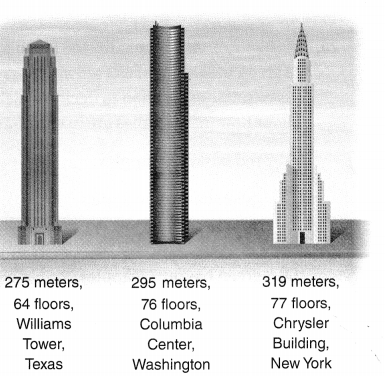Question 15. About how many meters tall is each floor of the Chrysler Building? Answer: The given figure is:Now, From the given figure, We can observe that The Chrysler Building is 319 meters and has 77 floors Now, The height of each floor in the Chrysler Building = 319 ÷ 77 Now, Estimate: 320 ÷ 80 = 4 240 ÷ 80 = 3 Now, Since 320 is close to 319, the estimate of 320 will be more reasonable Hence, from the above, We can conclude that The height of each floor in the Chrysler Building is about 4 meters Question 16. H.O.T. Multi-Step Use estimation to decide which building has the tallest floors. About how many meters is each floor?Answer: The given figure is:Now, From the given figure, We can observe that The height of the Williams Tower is: 275 meters The height of the Columbia Center is: 295 meters The height of the Chrysler Building is: 319 meters Now, The height of each floor in the Williams Tower = 275 ÷ 64 The height of each floor in the Columbia Center = 295 ÷ 76 The height of each floor in the Chrysler Building = 319 ÷ 77 Now, Estimate: The height of each floor in the Williams Tower = 280 ÷ 70 = 4 meters The height of each floor in the Columbia Center = 320 ÷ 80 = 4 meters The height of each floor in the Chrysler Building = 320 ÷ 80 = 4 meters Hence, from the above, We can conclude that The Chrysler Building is the tallest The floors in each building are about 4 meters Question 17. H.O.T. Eli needs to save$235. He plans to mow lawns and charge $21 for each. Write two estimates for the number of lawns he needs to mow. Decide which estimate you think is the better one for Eli to use. Explain your reasoning. Answer: It is given that Eli needs to save$235. He plans to mow lawns and charge $21 for each. Write two estimates for the number of lawns he needs to mow Now, According to the given situation, The number of lawns Eli needs to mow =$235 ÷ $21 Now, Estimate:$240 ÷ $20 = 12$200 ÷ $20 = 10 Now, Since$240 is close to $235, the estimate of$240 will be more reasonable
Hence, from the above,
We can conclude that
The number of lawns Eli needs to mow is about 12 lawns

Question 18.
A meteoroid travels 18 miles per second and is 2,863 miles away from the moon. Estimate how long it will take the meteoroid to reach the moon.
(A) 120 seconds
(B) 150 seconds
(C) 200 seconds
(D) 280 seconds
It is given that
A meteoroid travels 18 miles per second and is 2,863 miles away from the moon
Now,
The distance that it took for the meteoroid to reach the moon = 2,863 ÷ 18
Now,
Estimate:
3,000 ÷ 20 = 150
2,800 ÷ 20 = 140
Hence, from the above,
We can conclude that
The distance that it took for the meteoroid to reach the moon is about: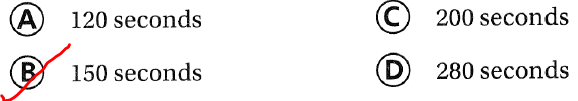Question 19.
Apply A playground is 5,583 feet away from Jake’s house. Jake runs 17 feet per second. Which is the best estimate of the amount of time it will take Jake to run to the park?
(A) 5,000  ÷ 20 = 260 seconds
(B) 6,000  ÷ 20 = 300 seconds
(C) 6,000  ÷ 10 = 600 seconds
(D) 5,000  ÷ 10 = 500 seconds
It is given that
A playground is 5,583 feet away from Jake’s house. Jake runs 17 feet per second
Now,
According to the given information,
The amount of time it will take Jake to run to the park = 5,583 ÷ 17
Now,
Estimate:
5,600 ÷ 20 = 280
6,000 ÷ 20 = 300
Hence, from the above,
We can conclude that
The amount of time it will take Jake to run to the park is about:Question 20.
Multi-Step At an orchard, 486 green apples are to be organized into 12 green baskets, and 633 red apples are to be organized into 31 red baskets. Use estimation to decide which color basket has more apples. About how many apples are in each basket of that color?
It is given that
At an orchard, 486 green apples are to be organized into 12 green baskets, and 633 red apples are to be organized into 31 red baskets
Now,
According to the given information,
The number of green apples organized in each basket = 486 ÷ 12
The number of red apples organized in each basket = 633 ÷ 31
Now,
Estimate:
The number of green apples organized in each basket = 500 ÷ 10
= 50 green apples
The number of red apples organized in each basket = 630 ÷ 30
= 21 red apples
Hence, from the above,
We can conclude that
The color basket that has more apples and the number of apples in each basket of that color is about: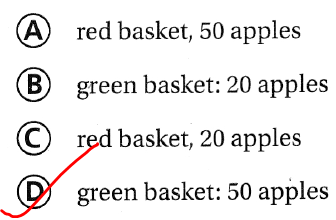Texas Test Prep

Question 21.
Anik built a tower of cubes. It was 594 millimeters tall. The height of each cube was 17 millimeters. About how many cubes did Anik use?
(A) 10
(B) 300
(C) 30
(D) 16
It is given that
Anik built a tower of cubes. It was 594 millimeters tall. The height of each cube was 17 millimeters.
Now,
According to the given information,
The number of cubes did Anik used = 594 ÷ 17
Now,
Estimate:
The number of cubes did Aik used = 600 ÷ 20
= 30 cubes
Hence, from the above,
We can conclude that
The number of cubes did Anik used is about:### Texas Go Math Grade 5 Lesson 2.5 Homework and Practice Answer Key

Use compatible numbers to find two estimates.

Question 1.The given division expression is: 396 ÷ 42
Now,
Estimate:
400 ÷ 40                    (or)            360 ÷ 40
= 10                             (or)           = 9
Now,
Since 396 is close to 400, the estimate of 400 will be more reasonable
Hence, from the above,
We can conclude that
The value of the given expression is about 10

Question 2.The given division expression is: 413 ÷ 59
Now,
Estimate:
420 ÷ 60                    (or)            4360 ÷ 60
= 7                             (or)            = 6
Now,
Since 420 is close to 413, the estimate of 420 will be more reasonable
Hence, from the above,
We can conclude that
The value of the given expression is about 7

Question 3.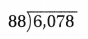The given division expression is: 6,078 ÷ 88
Now,
Estimate:
6,300 ÷ 90                    (or)            5,400 ÷ 90
= 70                             (or)            = 60
Now,
Since 6,300 is close to 6,078, the estimate of 6,300 will be more reasonable
Hence, from the above,
We can conclude that
The value of the given expression is about 70

Use compatible numbers to estimate the quotient.

Question 4.
7,233 ÷ 84
The given division expression is: 7,233 ÷ 84
Now,
Estimate:
7,200 ÷ 80                    (or)            8,000 ÷ 80
= 90                             (or)            = 100
Now,
Since 7,200 is close to 7,233, the estimate of 7,200 will be more reasonable
Hence, from the above,
We can conclude that
The value of the given expression is about 90

Question 5.
568 ÷ 34
The given division expression is: 568 ÷ 34
Now,
Estimate:
540 ÷ 30                    (or)            570 ÷ 30
= 18                           (or)            = 19
Now,
Since 568 is close to 5700, the estimate of 570 will be more reasonable
Hence, from the above,
We can conclude that
The value of the given expression is about 19

Question 6.
938 ÷ 57
The given division expression is: 938 ÷ 57
Now,
Estimate:
900 ÷ 60                    (or)            900 ÷ 50
= 15                             (or)            = 18
Now,
Since 938 is close to 900, the estimate of 900 will be more reasonable
Hence, from the above,
We can conclude that
The value of the given expression is about 15

Question 7.
4,479 ÷ 89
The given division expression is: 4,479 ÷ 89
Now,
Estimate:
4,500 ÷ 90                    (or)            3,600 ÷ 90
= 50                             (or)            = 40
Now,
Since 4,479 is close to 4,500, the estimate of 4,500 will be more reasonable
Hence, from the above,
We can conclude that
The value of the given expression is about 50

Question 8.
1,238 ÷ 57
The given division expression is: 1,238 ÷ 57
Now,
Estimate:
1,200 ÷ 60                    (or)            900 ÷ 60
= 20                             (or)            = 15
Now,
Since 1,238 is close to 1,200, the estimate of 1,200 will be more reasonable
Hence, from the above,
We can conclude that
The value of the given expression is about 20

Question 9.
5,587 ÷ 77
The given division expression is: 5,587 ÷ 77
Now,
Estimate:
5,600 ÷ 80                    (or)            4,800 ÷ 80
= 70                             (or)            = 60
Now,
Since 5,587 is close to 5,600, the estimate of 5,600 will be more reasonable
Hence, from the above,
We can conclude that
The value of the given expression is about 70

Question 10.
4,192 ÷ 55
The given division expression is: 4,192 ÷ 55
Now,
Estimate:
4,200 ÷ 60                    (or)            3,600 ÷ 60
= 70                             (or)            = 960
Now,
Since 4,192 is close to 4,200, the estimate of 4,200 will be more reasonable
Hence, from the above,
We can conclude that
The value of the given expression is about 70

Question 11.
1,847 ÷ 24
The given division expression is: 1,847 ÷ 24
Now,
Estimate:
1,500 ÷ 25                    (or)            2,000 ÷ 525
= 60                             (or)            = 80
Now,
Since 1,847 is close to 2,000, the estimate of 2,000 will be more reasonable
Hence, from the above,
We can conclude that
The value of the given expression is about 60

Problem Solving

Question 12.
Alec needs to save $376. He plans to rake fall leaves for S12 per lawn. Using compatible numbers, write an estimate that shows the number of lawns he will need to rake. Answer: It is given that Alec needs to save$376. He plans to rake fall leaves for S12 per lawn
Now,
According to the given information,
The number of lawns Alec will need to make = $376 ÷$12
Now,
Estimate:
The number of lawns Alec will need to make = $360 ÷$12
= 30
The number of lawns Alec will need to make = $480 ÷$12
= 40
Hence, from the above,
We can conclude that
The number of lawns Alec will need to make is about 30 to 40 lawns

Question 13.
Mr. Rodriguez’s construction company built an office building that is 1,848 feet tall. Each floor of the building is 14 feet high. About how many floors are in the building? Write an estimate.
It is given that
Mr. Rodriguez’s construction company built an office building that is 1,848 feet tall. Each floor of the building is 14 feet high
Now,
According to the given information,
The number of floors that are present in the building = 1,848 ÷ 14
Now,
Estimate:
The number of floors that are present in the building = 1,600 ÷ 16
= 100
The number of floors that are presen in the building = 2,400 ÷ 16
= 150
Hence, from the above,
We can conclude that
The number of buildings that are in the building is about 100 to 150 buildings

Lesson Check

Question 14.
Which number sentence with compatible numbers is the most reasonable estimate of the quotient for 478 ÷ 47?
(A) 500 ÷ 25 = 20
(B) 500 ÷ 50 = 10
(C) 400 ÷ 50 = 8
(D) 600 ÷ 40 = 15
The given division expression is: 478 ÷ 47
Now,
Estimate:
500 ÷ 50 = 10
Hence, from the above,
We can conclude that
The number sentence with compatible numbers that is the most reasonable estimate of the quotient for 478 ÷ 47 is: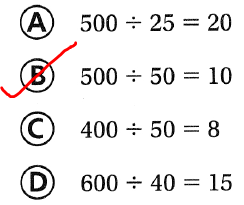Question 15.
Cameron wants to buy a bike for $268. He saves$53 each month from his job. Which is the best estimate of the number of months it will take him to save enough to buy the bike?
(A) 150 ÷ 50 = 3months
(B) 300 ÷ 60 = 5 months
(C) 300 ÷ 50 = 6 months
(C) 240 ÷ 60 = 4months
It is given that
Cameron wants to buy a bike for $268. He saves$53 each month from his job
Now,
According to the given information,
The number of months it will take Cameron to save enough money to buy the bike = $268 ÷$53
Now,
Estimate:
The number of months it will take Cameron to save enough money to buy the bike = $250 ÷$50
= 5 months
The number of months it will take Cameron to save enough money to buy the bike = $300 ÷$50
= 6 months
Hence, from the above,
We can conclude that
The number of months it will take Cameron to save enough money to buy the bike is about: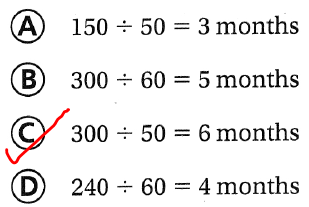Question 16.
Leo has 279 comic books in his collection. He puts 34 comic books in each box. About how many boxes of comic books does Leo have?
(A) 6
(B) 11
(C) 9
(D) 5
It is given that
Leo has 279 comic books in his collection. He puts 34 comic books in each box
Now,
According to the given information,
The number of boxes of comic books does Leo have = 279 ÷ 34
Now,
Estimate:
The number of boxes of comic books does Leo have = 270 ÷ 30
= 9 boxes
Hence, from the above,
We can conclude that
The number of boxes of comic books does Leo have is about: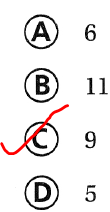Question 17.
The Alam family has saved $260 to spend on entertainment activities for one week. They want to spend about the same amount of money each day. Which is the best estimate of how much they can spend each day? (A)$280 ÷ 7 = $40 (B)$260 ÷ 10 = $26 (C)$240 ÷ 6 = $40 (D)$270 ÷ 9 = $30 Answer: It is given that The Alam family has saved$260 to spend on entertainment activities for one week. They want to spend about the same amount of money each day
Now,
We know that,
1 week = 7 days
Now,
According to the given information,
The amount of money the Alam family spend each day = $260 ÷ 7 Now, Estimate: The amount of money the Alam family spend each day =$280 ÷ 7
= \$40
Hence, from the above,
We can conclude that
The amount of money the Alam family spend each day is about: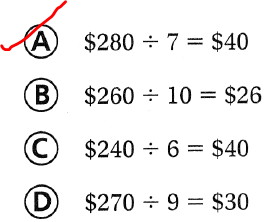Question 18.
Multi-Step At the recycling center, 394 cans are to be organized into 10 green bins, and 560 bottles are to be organized into 12 blue bins. Use estimation to decide which type of bin has more items. About how many items are in this bin?
(A) blue bin, 60
(B) blue bin, 40
(C) green bin, 60
(D) green bin, 40
It is given that
At the recycling center, 394 cans are to be organized into 10 green bins, and 560 bottles are to be organized into 12 blue bins
Now,
According to the given information,
The number of cans present in each green bin = 394 ÷ 10
The number of cans present in each blue bin = 560 ÷ 12
Now,
Estimate:
The number of cans present in each green bin = 400 ÷ 10
= 40 cans
The number of cans present in each blue bin = 600 ÷ 10
= 60 cans
Hence, from the above,
We can conclude that
The type of bin that has more items and the number of  items that are in this bin is:Question 19.
Multi-Step Farmer Theo sells cases of chicken eggs with 30 dozen eggs in a case. If he has 9,370 eggs, how many cases of eggs does he have?
(A) 20
(B) 17
(C) 30
(D) 35
It is given that
Farmer Theo sells cases of chicken eggs with 30 dozen eggs in a case and he has 9,370 eggs
Now,
According to the given information,
The number of cases of eggs that Theo has = 9,370 ÷ (30 × 12)
= 9,370 ÷ 360
= 26.9
≅ 30 cases
Hence, from the above,
We can conclude that
The number of cases of eggs that Theo has is:Scroll to Top